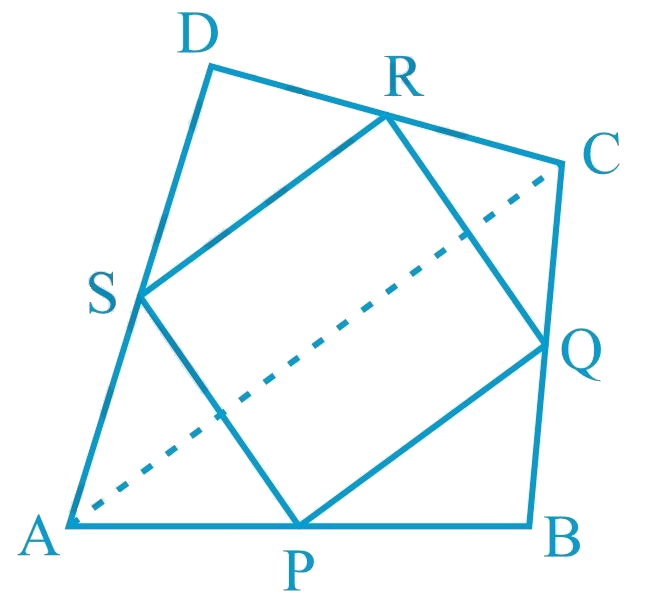# Ex.8.2 Q1 Quadrilaterals Solution - NCERT Maths Class 9

Go back to  'Ex.8.2'

## Question

$$ABCD$$ is a quadrilateral in which $$P$$, $$Q$$, $$R$$ and $$S$$ are mid-points of the sides $$AB$$, $$BC$$, $$CD$$ and $$DA$$ (see the given figure). $$AC$$ is a diagonal. Show that:i) \begin{align}{SR}\; \| \; {AC} \; \rm{and} \; {SR}=\frac{1}{2} {AC} \end{align}

ii) $$PQ = SR$$

iii) $$PQRS$$ is a parallelogram.

Video Solution
Ex 8.2 | Question 1

## Text Solution

What is known?

$$ABCD$$ is a quadrilateral in which $$P, Q, R$$ and $$S$$ are mid-points of the sides $$AB, BC, CD$$ and $$DA. AC$$ is a diagonal.

What is unknown?

How we can show that

(i) $$SR\; AC$$ and $$SR \;AC$$

(ii) $$PQ SR$$

(iii) $$PQRS$$ is a parallelogram.

Reasoning:

In a triangle, the line segment joining the mid-points of any two sides of the triangle is parallel to the third side and is half of it. Also, if one pair of opposite sides of quadrilateral is parallel and equal to each other then it is a parallelogram.

Steps:

(i) In $$\Delta$$$$ADC$$, $$S$$ and $$R$$ are the mid-points of sides $$AD$$ and $$CD$$ respectively. In a triangle, the line segment joining the mid-points of any two sides of the triangle is parallel to the third side and is half of it.

\begin{align}\therefore SR \parallel AC \;and \; SR\!=\!\frac{1}{2}\ AC\quad\!\!... (1)\end{align}

(ii) In $$\Delta$$$$ABC$$, $$P$$ and $$Q$$ are mid-points of sides $$AB$$ and $$BC$$ respectively. Therefore, by using mid-point theorem,

\begin{align} PQ \parallel AC\; and \ PQ \; \!=\!\!\frac{1}{2} AC\quad\!\!... (2)\end{align}

Using Equations ($$1$$) and ($$2$$), we obtain$$PQ \; \| \;SR$$ and $$PQ = SR$$.. (3)

\begin{align} \therefore \rm PQ = SR \end{align}

(iii) From Equation ($$3$$), we obtained $$PQ$$ $$\parallel$$ $$SR$$ and $$PQ =SR$$

Clearly, one pair of opposite sides of quadrilateral $$PQRS$$ is parallel and equal.

Hence, $$PQRS$$ is a parallelogram.

Video Solution